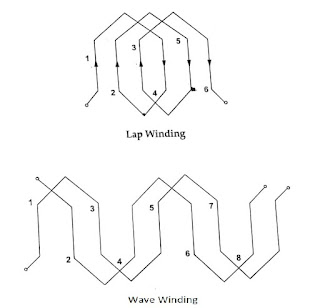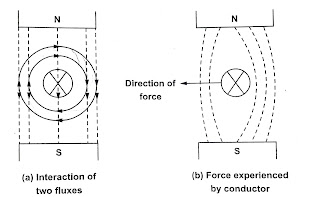Generators work on the principle of dynamically induced emf and faraday’s law of electro magnetic induction. It states that whenever there is a change in flux linking with a coil or conductor an electro motive force (emf) is induced in that coil or conductor. The relative motion can be achieved by rotating conductor with respect to flux or by rotating flux with respect to the conductor.

Such an induced electro motive force which is due to physical movement of coil or conductor with respect to flux or movement of flux with respect to coil or conductor is called dynamically induced emf. In practical generator when a d.c supply is given to the field winding which is wound on the poles, the magnetic flux is produced which is stationary. The number of conductors are connected in a specific manner to form a winding called armature winding of a d.c machine.

The part on which the winding is kept is called armature of d.c machine. This armature is rotated with the help of an external device called prime mover which is coupled. Thus the rotating armature cuts the magnetic flux produced in the field winding and an electro motive force (emf) is produced in the armature winding according to faraday’s law of electro magnetic induction. The direction of the induced emf is obtained from Fleming’s right hand rule. The magnitude of the induced electro motive force is given by

E =B x l x V

B = Flux density in Wb.

l = Active length of conductor in m

V = Relative velocity component of conductor in m/s in the direction perpendicular to direction of flux.

Case(i)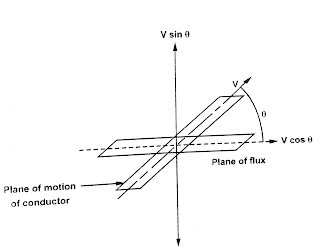Angle between the plane of motion of conductor and plane of flux in 6, then the induced emf at case i is given by E=B x Lx (V sin Θ )

where V sin Θ is the component perpendicular to plane of flux which is responsible for induced electro motive force.

Case (ii)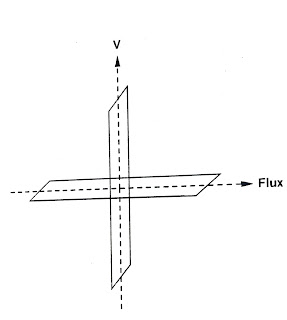The plane of motion of conductor is perpendicular to plane of flux so angle 0 – 90º. The induced electro motive force is given by

E=B x l x (V sin 90°)

E =B x l x V

The maximum electro motive force is attained at this case.

Case (iii)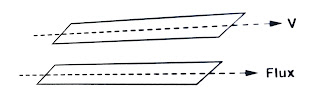The plane of motion of conductor is parallel to the plane of flux so angle 0 = 0°. The induced electro motive force is given by

E=B x l x (V sin 0)

E=0

From the three cases, the induced emf in a de generator is purely sinusoidal (i.e) alternating. To obtain d.c voltage, a device is used in a d.c generator to convert alternating electro motive force to unidirectional electro motive force (emf). This device is called commutator. This unidirectional emf is taken out with the help of brush which is the stationary part of d.c generator and is gives to the external circuit.

See More:

See More: Four and Two Point Starter

See More: Three Point Starter